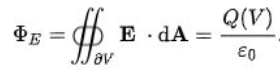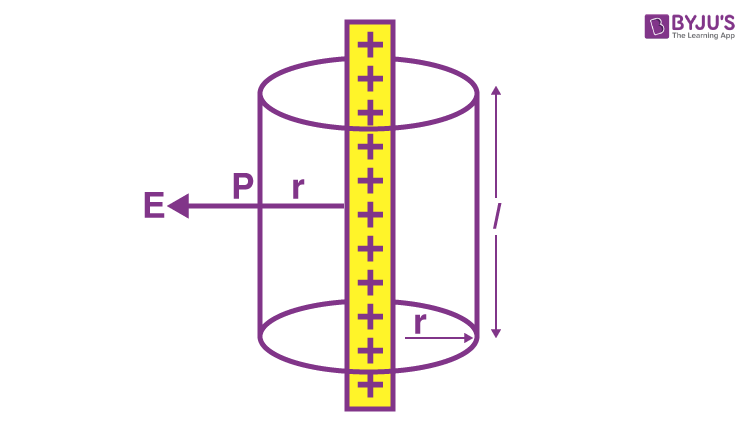# Electric Field Due To An Infinitely Long Charged Wire

In previous sessions, we have studied in detail about the electric field as well as the electric charges. We can calculate the electric field for any Gaussian surface. In this session, let us learn to calculate electric field due to infinite line charge or electric field due to an infinitely long straight uniformly charged wire.

## What is Electric Field?

An electric field is defined as the electric force per unit charge. It is given as:

E = F/Q

Where,

• E is the electric field
• F is the force
• Q is the charge

The variations in the magnetic field or the electric charges are the cause of electric fields. Volt per meter (V/m) is the SI unit of the electric field.

## What is Gaussian Surface?

A Closed Surface in a three-dimensional space whose flux of a vector field is calculated, which can either be the magnetic field or the electric field or the gravitational field, is known as the Gaussian Surface. Let us learn how to calculate electric field due to infinite line charge.## Electric Field Due To An Infinitely Long Straight Uniformly Charged Wire

Let us learn how to calculate electric field due to infinite line charge. Consider an infinitely long straight uniformly charged wire. Let the linear charge density of this wire be λ. P is the point that is located at a perpendicular distance from the wire. The distance between point P and the wire is r.The wire is considered to be a cylindrical Gaussian surface. This is because to determine the electric field

$$\begin{array}{l}\vec{E}\end{array}$$
at point P Gauss law is used.

The surface area of the curved part is given as:

S = 2πrl

The total charge enclosed by the Gaussian surface is given as:

q = λl

The electric flux through the end surfaces of the cylindrical Gaussian surface is given as:

Φ1 = 0

The electric flux through the curved surface of the cylindrical Gaussian surface is given as:

Φ2 = E cosθ.s

Φ2 = E x 1 x 2πrl

The total electric flux is given as:

Φ = Φ1 + Φ2

Φ = 0 + E cosθ.s

Φ2 = 2πrlE (eq. 1)

From Gauss law, we know that

$$\begin{array}{l}\varphi =\frac{q}{\epsilon _{o}}=\frac{\lambda l}{\epsilon_{o}}\end{array}$$
(eq.2)

From eq 1. And eq 2

2πrlE =

$$\begin{array}{l}\frac{\lambda l}{\epsilon_{o}}\end{array}$$

$$\begin{array}{l}E=\frac{1}{2\pi \epsilon _{o}}\frac{\lambda }{r}\end{array}$$

Therefore, the above equation is the electric field due to an infinitely long straight uniformly charged wire.

Learn about the characteristics of electrical force with the help of the video below.Hope you have learned about electric field due to infinite line charge or electric field due to an infinitely long straight uniformly charged wire. Stay tuned with BYJU’S to learn more about other concepts.

## Frequently Asked Questions – FAQs

### What is the Gaussian surface of a sphere?

The following equation is the Gaussian surface of a sphere:

$$\begin{array}{l}E=\frac{Q_{A}}{4\pi \epsilon _{o}r^{2}}\end{array}$$

### What is the Gaussian surface of a cylinder?

The following equation is the Gaussian surface of a cylinder:

$$\begin{array}{l}E=\frac{\lambda }{2\pi \epsilon _{o}r}\end{array}$$

### Can an electric field be zero?

Yes, an electric field can be zero. For unlike charges, the electric field is zero outside when the magnitude of the charge is small. For like charges, the electric field is zero along the line joining the two charges.

### At which point is the electric field the weakest?

We know that the electric field is proportional to the density of the field lines. Therefore, when the field lines are far, the electric field is the weakest.

### What direction do electric fields travel?

The direction of the electric fields is the same as the direction of the electrical force that is acting on the positive charge.